# Random Forests

## Decision Trees

Pros

• Interpretable
• Simple
• Classification or Regression

Cons

• Poor accuracy
• High variance

## Bias vs Variance$Error = Variance + Bias^2 + noise$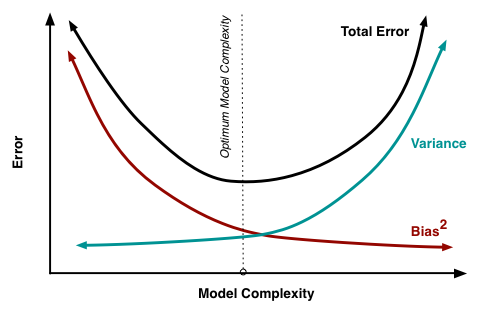Reduce bias: Build more complex models

Reduce variance: Use a lot of data or simple model

How to reduce the error caused by noise?

## Fisher Rolling in his Grave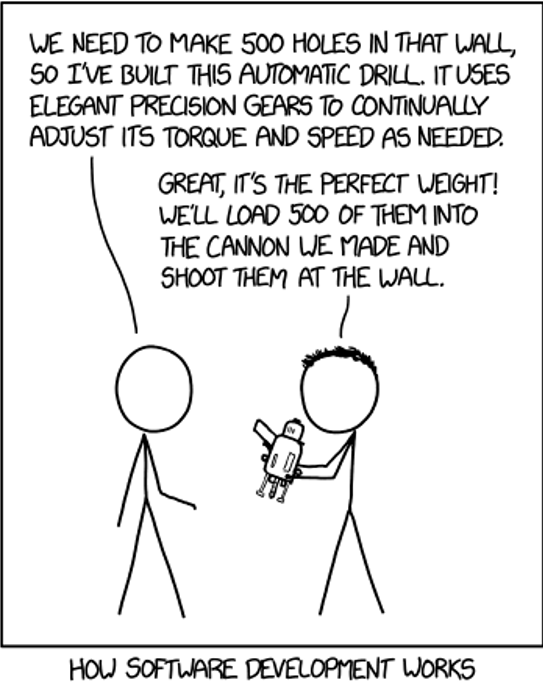## Bagging

Averaging a set of observations reduces variance.

But we only have one training set ... or do we?## Bootstrap

Simulate new datasets:

Take samples (with replacement) from original training set

Repeat $n$ times## Bootstrap visualization

Sample has $n$ elements.

Probability of getting picked: $\frac{1}{n}$

Probability of not getting picked: $1-\frac{1}{n}$

If you sample $n$ elements with replacement, the probability for each element of not getting picked in the sample is: $(1-\frac{1}{n})^n$

As ${n\to\infty}$, this probability approaches $\frac{1}{e}\approx.368$

Thus, $0.632$ of the data points in your original sample show up in the Bootstrap sample (the other $0.368$ won't be present in it)

## Bagging

Train a tree on each bootstrap sample, and average their predictions (Bootstrap Aggregating)

## Random Forests

Like bagging, but removes correlation among trees.

At each split, considers only a subset of predictors.Notes:
Random forests typically consider $\sqrt{\textrm{number of features}}$ or $1/3$ at each split (if 10 features, then it considers ~3 features), and picks the best one.

Slide credit G. Calmattes

## Random ForestsSlide credit G. Calmattes

### A Step away from Interpretation

• How do you model a vote from many trees?
• Improved prediction, reduced interpretation
• Feature importance
• Mean decrease in Gini index relative to the max
• Show’s influence not directionality
• Bucketing importance on highly dimensional data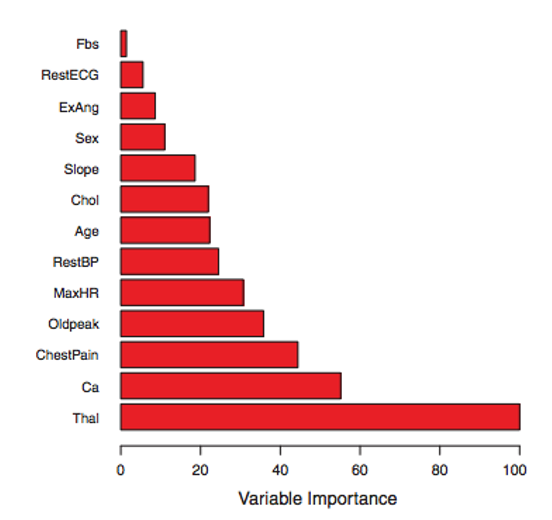## Hyperparameter Selection

What is a hyperparameter?

Which dataset should we use to select hyperparameters? Train? Test?

## Validation Dataset

Split the dataset into three!

• Train on the training set
• Select hyperparameters based on performance of the validation set
• Test on test set

What if you don't have enough data to split in 3 separate sets?

## K-Fold Cross Validation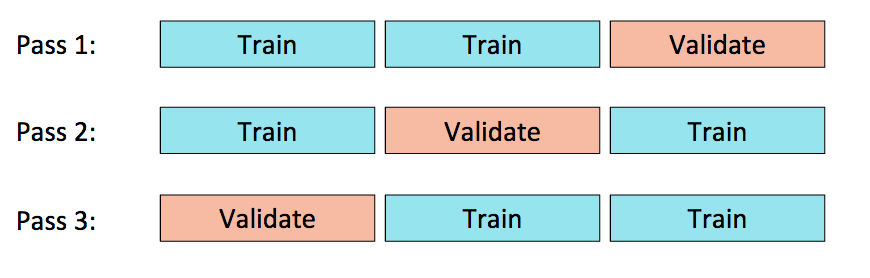## Grid Search

Try various hyperparameter settings to find the optimal combination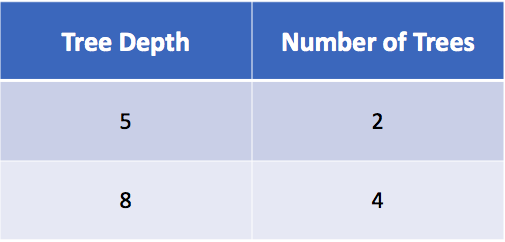How many models will this build (k = 3)?

## Problems with Grid Search

Exhaustive enumeration is expensive

You have to determine the exact search space

Past information on good hyperparameters isn’t used

So what if...

• You have a training budget
• You have a lot of hyperparameters to tune
• Want to pick your hyperparameters based on past results

## Hyperopt

Optimization over awkward search spaces

Serial and parallel

Spark integration

3 algorithms

• Random Search
• Tree of Parzen Estimators (TPE)

## Random Search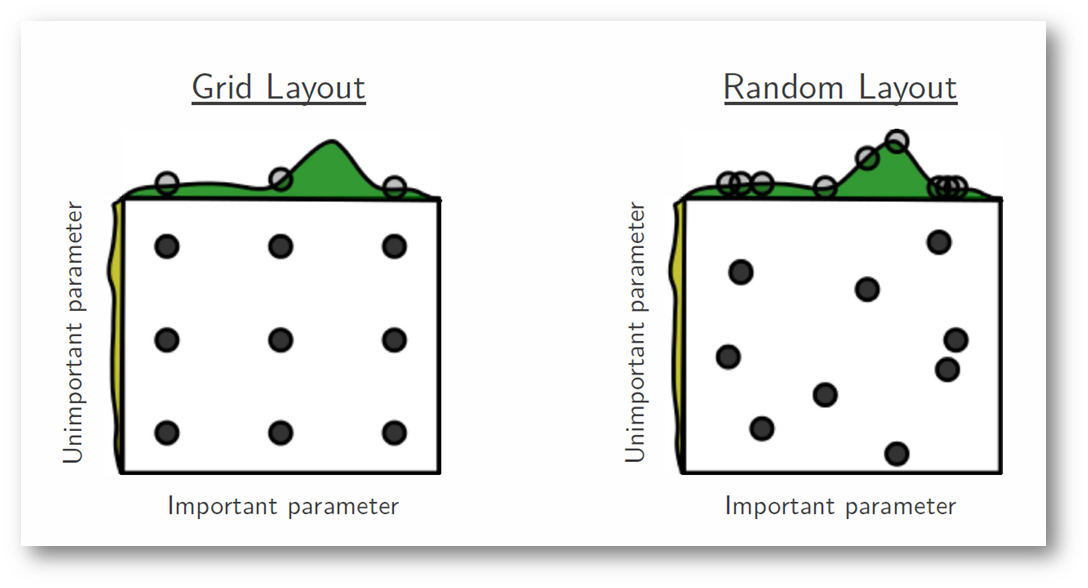Generally outperforms exhaustive search

Can struggle with on some datasets (e.g. convex spaces)

## Tree of Parzen Estimators

Meta-learner, Baysian process

Non-parametric densities

Returns candidate hyperparameters based on best Expected Improvement (EI)

Provide a range and distribution for continuous and discrete values

Adaptive TPE better tunes the search space (e.g. freezes hyperparameters, tunes # random trials before TPE)

## Other Ensemble Models

Sequential method

Fit trees to residuals (on first iteration, residuals are the true predictions)

Idea: build tree more slowly

These trees are NOT independent of each other

## GBDT

 Y Prediction Residual $40$ $35$ $5$ $60$ $67$ $-7$ $30$ $28$ $2$ $33$ $32$ $1$ $25$ $27$ $-2$
 Y Prediction Residual $5$ $3$ $2$ $-7$ $-4$ $-3$ $2$ $3$ $-1$ $1$ $0$ $1$ $-2$ $-2$ $0$

 Y Prediction $40$ $38$ $60$ $63$ $30$ $31$ $33$ $32$ $25$ $25$

## Boosting vs Random Forest

Boosting starts with high bias, low variance and works rightRF starts with high variance and reduces it by averaging many trees

## Kaggle

Data Science and Machine Learning Competition site

XGBoost one of most winning solutions

https://www.kaggle.com/

## What is XGBoost?

Boosted trees with…

• Weighted quantile sketch for approximative learning
• Smart cache access and data compression
• A sparsity-aware algorithm for sparse data
• Sharding

10x speed increase

Scale in the billions

Parallelized and distributed